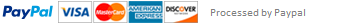### Python Temperature Converter

You are to develop a program that the user will enter a group of temperatures to be processed. First, you must ask the user if the temperatures they are entering are in Fahrenheit or Celsius format.

You will need to convert the temperatures to the opposite format. Then you add both temperatures to a total for each group. The number of temperatures to enter will be eight. Once you have completed entering all eight temperatures.

You will calculate the average for both Fahrenheit and Celsius which will be printed out. The conversion formulae are: F = 1.8 * C + 32 to convert Celsius to Fahrenheit and C = (F - 32) * 5/9 to convert Fahrenheit to Celsius. Create an algorithm, flowchart/s, and pseudocode.

This assignment has been answered 5 times in private sessions.